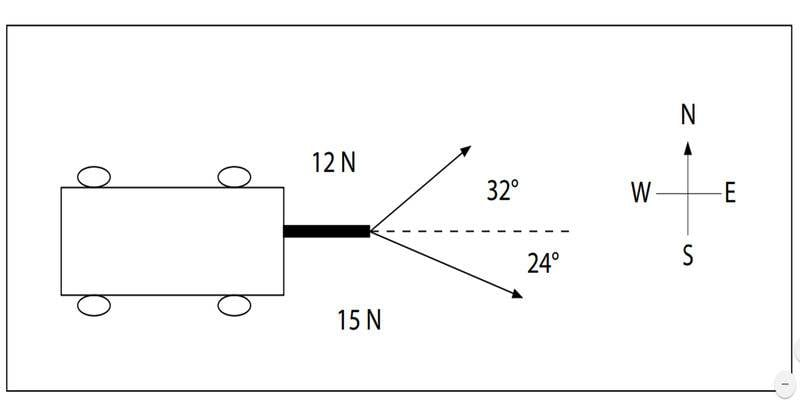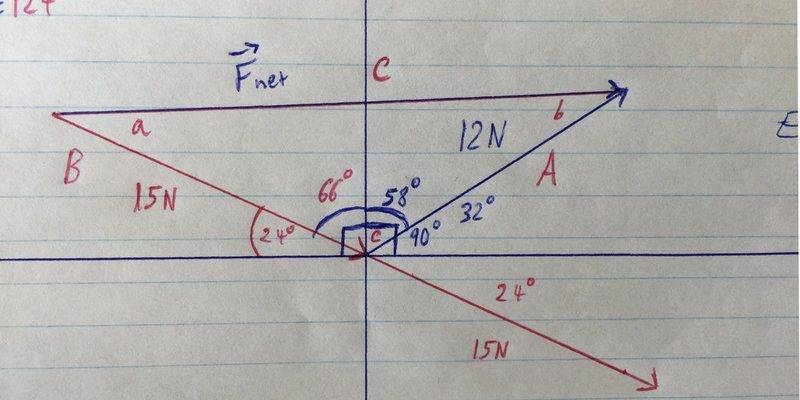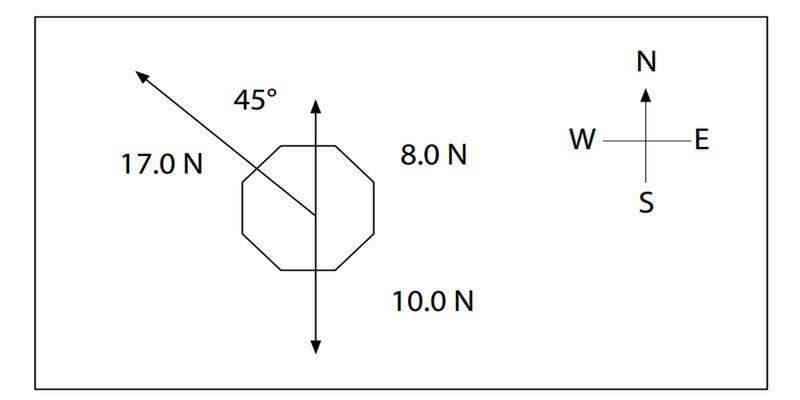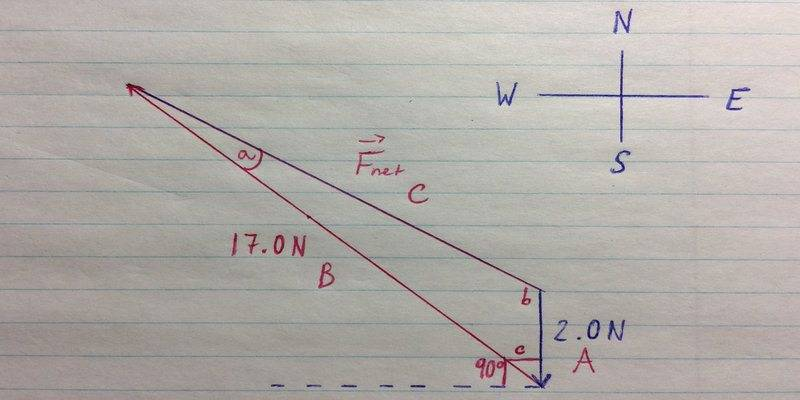# Calculating the net force acting on an object from a diagram

Humbleness

## Homework Statement

Calculate the net force acting on the object in the following diagram:## Homework Equations

cosine law / sine law
ONLY solving using cosine/sine laws.

## The Attempt at a SolutionFirst using the cosine law to find the magnitude of the net force:
c2=a2+b2-2abcosC
c2=122+152-2 x 12 x 15 (cos124)
c2=369-(-201)
c2=570
c=23.9N

Now using sine law to find the angle:
sina/12=sinb/15=sin124/23.9
b=sin-1 (sin124/23.9 x 15)
b=31 degrees

Now I just don't know in which direction am I supposed to say it is pointing towards? Because if you look at the diagram and my work, it looks as if it is almost straight East. What should I do?

## Answers and Replies

Homework Helper
Gold Member
2021 Award
ONLY solving using cosine/sine laws.
Are you saying that you are asked to find the direction of the resultant using the laws of sines and cosines only? If so, it looks like the resultant is pointing a certain number of degrees "North of East". What is that number? Hint: First find how big angle a is in your drawing.

•Humbleness
Humbleness
So I found for angle a using sine law:
sina/12N = sin31/15N
a = sin-1(sin31/15 x 12)
a = 24.3 degrees

I'm not sure what to do after this step, and honestly I don't believe I "have to" use only cosine, sine laws, however that is what I've been taught in the lesson. I got the exact same answer (23.9N) for the magnitude of the net force by solving the exact same way as in this video:
Which is basically just adding the vectors to get the sum, which is the net force.
But I'm pretty sure the answer would require a direction as well, no?
Maybe it isn't asking me of direction too; if I were to perfectly re-draw the diagram, wouldn't the net force be facing directly towards East, just like that middle dotted line in the original diagram?

The exact question, word-for-word is like this:

Calculate the net force acting on the object indicated in the following diagram. Show your work.

Homework Helper
Gold Member
2021 Award
a = 24.3 degrees
OK, and what is the angle that C makes above the horizontal (East) direction? That's the angle "North of East".
But I'm pretty sure the answer would require a direction as well, no?
Yes. Force is a vector and when you are asked to find a vector, you must supply magnitude and direction. If you were meant to supply the magnitude only, then there should be language in the problem to that effect.
... if I were to perfectly re-draw the diagram, wouldn't the net force be facing directly towards East, just like that middle dotted line in the original diagram?
No. I did the problem and the angle above the horizontal is small but not zero. I suggest that you redo the calculations to five decimals. You can always drop some in the end.

•Humbleness
Humbleness
Clever...
(Showing in the attachment that it's true, it isn't exactly going east.

I re-did the calculations with 5 decimals, as you suggested, and got:
Angle a = 24.597
Angle b = 31.353

And I found based entirely on using my protractor (Because I don't know what equation to use to calculate the angle from the horizontal ground), that the angle comes around roughly 0.2 degrees north of east.

#### Attachments

Homework Helper
Gold Member
2021 Award
Draw a horizontal line starting at the tail of vector B. The angle between this line and vector B is 24o. Do you see why?

•Humbleness
Humbleness
Ooohh, I see it now... That is why I was supposed to calculate by 5 decimals too, so that I come as close as possible to the actual result.
So by re-doing the calculations one more time, this time including everything (beyond just 5 decimals)
I get:
Angle a = 24.59825
Therefore the direction of the 23.9N net force will be 0.598 or better 0.6 degrees north of east.

Homework Helper
Gold Member
2021 Award
Therefore the direction of the 23.9N net force will be 0.598 or better 0.6 degrees north of east.
Yup, that's what I got.

•Humbleness
Humbleness
Yup, that's what I got.

Yey!Thank you so much for your help! Really, I appreciate it very much.

I just have one more solving net force from a diagram problem, which I already solved, and I would really appreciate confirmation if I got it correct, and if not, where I may have done wrong, if it's not too much trouble:Same equations, using cosine and sine laws to find both the magnitude and then angle and direction of the net force.

First I subtracted 10N and 8N in order to get:
-8.0N (S) + 10.0N (S) = 2.0N (S)

Then I was able to use tip-to-tail method:First using cosine law to find magnitude of net force:
c2=a2+b2-2abcosC
c2=4+289-2x2x17 (cos45)
c2=293-48
c=15.6N

Then angle:
sina/2N = sinb/17N = sin45/15.6N
b = sin-1 (sin45/15.6 x 17)
b = 50.4

Therefore the net force is 15.6N (S 50.4 W) West of South

#### Attachments

•